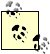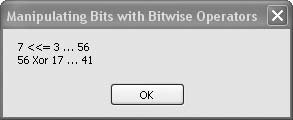# Recipe 6.24. Manipulating Bits with Bitwise Operators

#### Problem

You need to shift, mask, and perform other bitwise manipulations on integers.

#### Solution

Visual Basic 2005 has functions for all the major bit-manipulation techniques, and it's easy to combine these to perform more complicated bitwise calculations as required.

#### Discussion

There are several operators that are most often thought of as Boolean operators, working with and returning true and False (Boolean) values. However, these operators also accept and return integer values of various sizes, and this is where they can be of value for bit manipulations. These bitwise operators include the following:

And

Bits are combined to 1 only if they are both 1.

Not

Bits are inverted, 0 to 1 and 1 to 0.

Xor

Bits are combined to 1 only if the two bits are not the same.

Or

Bits are combined to 1 if either bit is a 1.

<<

Bits are all shifted left a given number of bit positions.

>>

Bits are all shifted right a given number of bit positions.The two bit-shift operators can be used as assignment operators. That is, the following two lines of code provide identical results: ` a = a << 3 a <<= 3 ` In both cases the bits in integer variable a are shifted to the left three positions. The And, Or, Not, and Xor operators don't support assignment notation.

The following code demonstrates a sampling of these bit manipulations. You can change the program to experiment with the various operators:

` Dim result As New System.Text.StringBuilder Dim number As Integer = 7 result.Append(number) result.Append(" <<= 3 … ") number <<= 3 result.AppendLine(number) result.Append(number) result.Append(" Xor 17 … ") number = number Xor 17 result.AppendLine(number) MsgBox(result.ToString()) `

Figure 6-24 shows the output displayed by this sample code.

##### Figure 6-24. Bit manipulations with Visual Basic 2005Visual Basic 2005 Cookbook: Solutions for VB 2005 Programmers (Cookbooks (OReilly))
ISBN: 0596101775
EAN: 2147483647
Year: 2006
Pages: 400

Similar book on Amazon